# NCERT Solutions For Class 10 Maths Chapter 13 Exercise 13.2

Go back to  'Surface Areas and Volumes'

## Chapter 13 Ex.13.2 Question 1

A solid is in the shape of a cone standing on a hemisphere with both their radii Being equal to $$1\,\rm{cm}$$ and the height of the cone is equal to its radius. Find the volume of the solid in terms of $$\pi$$.

### Solution

What is known?

A solid in the shape of a cone standing on a hemisphere. Radius and height of the cone are same as the radius of the hemisphere which is $$1\rm{cm}$$.

What is unknown?

The volume of the solid.

Reasoning:

Draw a figure of the solid to visualize it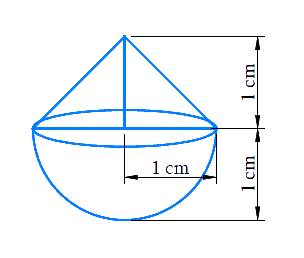Since the solid is made up of conical part and a hemispherical part.

Volume of the solid $$=$$ volume of the conical part $$+$$ volume of the hemispherical part

We will find the volume of the solid by using formulae;
Volume of the hemisphere  \begin{align} = \frac{2}{3}\pi {r^3}\end{align}
where $$r$$ is the radius of the hemisphere
Volume of the cone   \begin{align} = \frac{1}{3}\pi {r^2}h\end{align}
where $$r$$ and $$h$$ are the radius and height of the cone respectively.

Steps:

Radius of hemispherical part $$=$$ Radius of conical part $$=r=1\,\rm{cm}$$

Height of conical part $$= h = r = 1 \rm cm$$

Volume of the solid  $$=$$ volume of the conical part $$+$$ volume of the hemispherical part

\begin{align}&= \frac{1}{3}\pi {r^2}h + \frac{2}{3}\pi {r^3}\\&= \frac{1}{3}\pi {r^3} + \frac{2}{3}\pi {r^3}\\&= \pi {r^3}\\ &= \pi {\left( {1 \rm cm} \right)^3}\\&= \pi \rm c{m^3}\end{align}

## Chapter 13 Ex.13.2 Question 2

Rachel, an engineering student, was asked to make a model shaped like a Cylinder with two cones attached at its two ends by using a thin aluminum Sheet. The diameter of the model is $$3\,\rm{cm}$$ and its length is $$12\,\rm{cm}$$. If each cone has a height of $$2\,\rm{cm}$$, find the volume of air contained in the model that Rachel made. (Assume the outer and inner dimensions of the model to be nearly the same.)

### Solution

What is known?

Rachel made a model of aluminum sheet shaped like a cylinder with $$2$$ cones attached to its $$2$$ ends.

Diameter of the model $$=3\,\rm{cm}$$

Length of the model $$= 12\,\rm{cm}$$

Height of each cone $$=2\,\rm{cm}$$

The internal and external dimensions of model are same.

What is unknown?

Volume of air contained in the model made by Rachel.

Reasoning:

Draw a figure according to given data to visualize the model.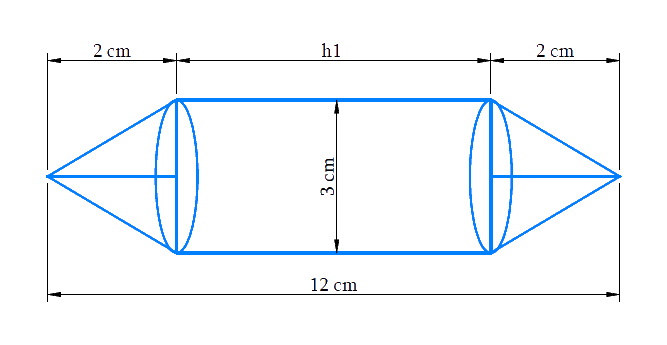From the figure it’s clear that the volume of the model includes volume of the cylindrical part and both the identical conical parts.

Volume of the model $$=$$ Volume of the $$2$$ conical parts $$+$$ volume of the cylindrical part

Hence, we work on the assumption that the outer and inner dimensions of the model are nearly the same.
Therefore, Volume of air in the model $$=$$ volume of the model based on outer dimensions

Since, length of the model includes height of the cylindrical part and height of both the identical conical parts and also diameter of the cylindrical part and conical parts are same as the diameter of the model.

Length of the model $$=$$ Height of the cylindrical part $$+ {\rm{ }}2 \times$$  Height of the conical part

We will find the volume of the model by using formulae;

Volume of the cylinder  $$= \pi {r^2}{h_1}$$

where $$r$$ and $$h_1$$ are the radius and height of the cylinder respectively.

Volume of the cone \begin{align} = \frac{1}{3}\pi {r^2}{h_2}\end{align}

where $$r$$ and $$h_2$$ are the radius and height of the cone respectively.

Steps:

Height of each conical part,  $${h_2} = 2 \rm cm$$

Height of cylindrical part $$=$$ Length of the model $$- {\rm{ }}2 \times$$ Height of the conical part

\begin{align}{h_1} &= 12 \rm cm - 2 \times 2cm\\&= 8cm\end{align}

Diameter of the model,  $$d = 3 \rm cm$$

Radius of cylindrical part $$=$$ radius of conical part \begin{align} = r = \frac{{3 \rm cm}}{2} = 1.5cm\end{align}

Volume of the model $$=$$ $$2 \times$$ Volume of the conical part $$+$$ volume of the cylindrical part

\begin{align}&= 2 \times \frac{1}{3}\pi {r^2}{h_2} + \pi {r^2}{h_1}\\&= \pi {r^2}\left( {\frac{2}{3}{h_2} + {h_1}} \right)\\&= \begin{bmatrix} \frac{{22}}{7} \times 1.5 \rm cm \times 1.5cm \\ \times \left( {\frac{2}{3} \times 2 \rm cm + 8cm} \right) \end{bmatrix} \\&= \begin{bmatrix} \frac{{22}}{7} \times 1.5 \rm cm \times \\ 1.5 \rm cm \times \frac{{28}}{3}cm \end{bmatrix} \\&= 66 \rm c{m^3}\end{align}

Volume of air in the model is $$66\,\rm{cm}^3.$$

## Chapter 13 Ex.13.2 Question 3

A Gulab jamun contains sugar syrup up to about $$30 \%$$ of its volume. Find approximately how much syrup would be found in $$45$$ Gulab jamuns, each shaped like a cylinder with two hemispherical ends with length $$5\,\rm{cm}$$ and diameter $$2.8\,\rm{cm}$$ (see Fig. 13.15).

### Solution

What is known?

Each Gulab jamun’s shape is like a cylinder with $$2$$ hemispherical ends with length $$5\rm{cm}$$ and diameter $$2.8\rm{cm.}$$
Each Gulab jamun contains sugar syrup up to about $$30\%$$ of its volume.

What is unknown?

Volume of sugar syrup in $$45$$ Gulab jamuns (approximately)

Reasoning:

Draw the figure to visualize the shape of Gulab jamun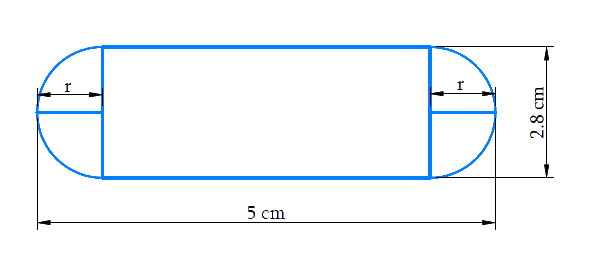Fig. 13.15

From the figure it’s clear that

Length of cylindrical part $$=$$ length of a Gulab jamun $$- {\rm{ }}2 \times$$ radius of the hemispherical part

Also,

Diameter of Gulab jamun $$=$$ diameter of cylindrical part

Radius of cylindrical part $$=$$ radius of hemispherical part

In order to find volume of sugar syrup in $$45$$ Gulab jamun we find volume of Gulab jamun

Using the statement

The volume of the solid formed by joining 2 basic solids will actually be sum of the volumes of its constituents.

Volume of $$1$$ Gulab jamun $$=$$ volume of cylindrical part $$+$$ volume of the $$2$$ hemispherical parts

Since, it’s given a Gulab jamun contains sugar syrup up to about $$30\%$$ of its volume. We make an assumption to every Gulab jamun contains sugar syrup at $$30\%$$ of its volume to simplify calculation

Volume of sugar syrup $$= 30\%$$ of volume of $$45$$ Gulab jamun.

Steps:

Diameter of the Gulab jamun,$$d = 2.8 \rm cm$$

Radius of cylindrical part $$=$$ radius of hemispherical part\begin{align} = r = \frac{{2.8 \rm cm}}{2} = 1.4cm\end{align}

Length of cylindrical part,$$h = 5 \rm cm - 2 \times 1.4cm = 2.2cm$$

Volume of $$1$$ Gulab jamun $$=$$ volume of cylindrical part$$+ {\rm{ 2}} \times$$ volume of the hemispherical parts

\begin{align}&= \pi {r^2}h + 2 \times \frac{2}{3}\pi {r^3}\\&= \pi {r^2}h + \frac{4}{3}\pi {r^3}\\&= \pi {r^2}\left( {h + \frac{4}{3}r} \right)\\&= \begin{bmatrix} \frac{{22}}{7} \times 1.4 \rm cm \times 1.4cm \\ \times \begin{pmatrix} 2.2 \rm cm + \frac{4}{3} \\ \times 1.4 \rm cm \end{pmatrix} \end{bmatrix} \\&= \begin{bmatrix} \frac{{22}}{7} \times 1.4 \rm cm \times \\ 1.4 \rm cm \times \frac{{122.2}}{3} \rm cm \end{bmatrix} \\&= \frac{{75.152}}{3} \rm c{m^3}\end{align}

Volume of $$45$$ Gulab jamuns $$= 45 \times$$ volume of $$1$$ Gulab jamun

\begin{align}&= 45 \times \frac{{75.152}}{3} \rm c{m^3}\\&= 15 \times 75.152 \rm c{m^3}\\&= 1127.28 \rm c{m^3}\end{align}

Volume of sugar syrup in $$45$$ Gulab jamuns$$= 30\%$$   of volume of $$45$$ Gulab jamun

\begin{align}&= \frac{{30}}{{100}} \times 1127.28 \rm c{m^{^3}}\\ &= 338.184 \rm c{m^3}\\ &= 338{\rm{ c{m^3 }}}({\text{approximately}})\end{align}

## Chapter 13 Ex.13.2 Question 4

A pen stand made of wood is in the shape of a cuboid with four conical depressions to hold pens. The dimensions of the cuboid are $$15 \,\rm{cm}$$ by $$10 \,\rm{cm}$$ by $$3.5 \,\rm{cm}$$. The radius of each of the depressions is $$0.5\,\rm{cm}$$ and the depth is $$1.4\,\rm{cm}$$.

Find the volume of wood in the entire stand (see Fig. 13.16).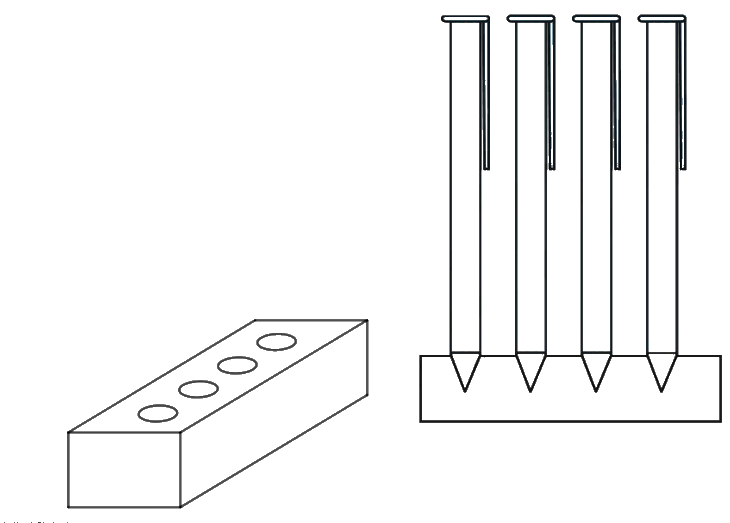Fig. 13.16

### Solution

What is known?

A wooden pen stand is in the shape of a cuboid with four conical depressions.

The dimensions of the cuboid are $$15\rm\,cm\; \times 10\,cm \;\times\; 3.5\,cm$$

Radius of conical depressions is $$\,0.5\,\rm{cm}$$

Depth of conical depression is $$1.4\,\rm{cm}$$.

What is unknown?

Volume of wood in the entire pen stand.

Reasoning:

From the given figure it’s clear that the conical depressions do not contain wood. Since the dimensions of all $$4$$conical depressions are the same, they will have identical volumes too.

Volume of wood in the entire pen stand $$=$$ volume of the wooden cuboid $$- {\rm{ }}4 \times$$volume of the conical depression

We will find the volume of the solid by using formulae;

Volume of the cuboid$$= lbh$$

where $$l, b$$ and $$h$$ are the length, breadth and height of the cuboid respectively.

Volume of the cone\begin{align} = \frac{1}{3}\pi {r^2}{h_1}\end{align}

where $$r$$ and $$h_1$$ are the radius and height of the cone respectively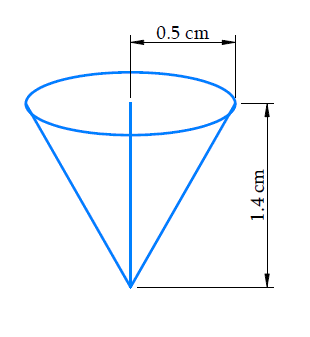Steps:

Depth of each conical depression,$${h_1} = 1.4 \rm cm$$

Radius of each conical depression, $$r = 0.5 \rm cm$$

Dimensions of the cuboid are $$15 \rm cm \times 10cm \times 3.5cm$$

Volume of wood in the entire pen stand $$=$$ Volume of the wooden cuboid $$- 4 \;\times$$ Volume of each conical depression

\begin{align}&= lbh - 4 \times \frac{1}{3}\pi {r^2}{h_1}\\ &=\!\left(15 \rm cm \!\times\! 10cm \!\times\! 3.5 \rm cm\!\right) -\left( 4\!\times\! \frac{1}{3} \!\times\! \frac{{22}}{7}\!\times\! 0.5 \rm cm \!\times\! 0.5cm \!\times\! 1.4 \rm cm \right) \\&= 525 \rm c{m^3} - 1.47c{m^3}\\&= 523.53 \rm c{m^3}\end{align}

## Chapter 13 Ex.13.2 Question 5

A vessel is in the form of an inverted cone. Its height is $$8\,\rm{cm}$$ and the radius of its top, which is open, is $$5\,\rm{cm}$$. It is filled with water up to the brim. When lead shots, each of which is a sphere of radius $$0.5\,\rm{cm}$$ are dropped into the vessel, one-fourth of the water flows out. Find the number of lead shots dropped in the vessel.

### Solution

What is known?

An open vessel is in the form of an inverted cone with height $$=8\,\rm{cm}$$ and radius of its top $$= 5\,\rm{cm}$$, which is filled with water up to the drum.

Spherical lead shots each of  radius $$=0.5\,\rm{cm},$$ are dropped into the vessel until then one-fourth of the water in the vessel flows out.

What is unknown?

Number of lead shots dropped into the vessel.

Reasoning:

Draw a figure of the vessel and lead shot to visualize it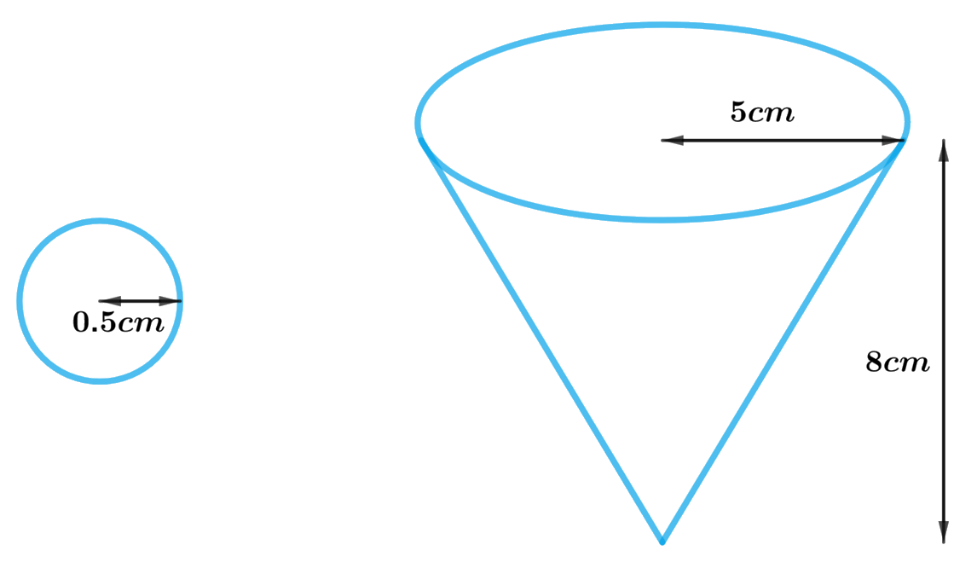Since the water is filled up to the brim in the vessel

Volume of water in the vessel $$=$$ volume of the conical vessel

Also, on dropping a certain number of lead shots (sphere) into the vessel one-fourth of the water flows out.

Volume of all lead shots dropped in the vessel \begin{align} = \frac{1}{4} \times \end{align}Volume of water in the vessel

Hence,

Number of lead shots \begin{align} = \frac{1}{4} \times\end{align}volume of the water in the vessel$$\div$$ volume of each lead shot

We will find the volume of the water in the vessel and lead shot by using formulae;

Volume of the sphere\begin{align} = \frac{4}{3}\pi {r^3}\end{align}

where $$r$$ is the radius of the hemisphere

Volume of the cone\begin{align}= \frac{1}{3}\pi {R^2}h\end{align}

where $$R$$ and $$h$$ are the radius and height of the cone respectively

Steps:

Height of the conical vessel,$$h = 8 \rm cm$$

Radius of the conical vessel,$$R = 5 \rm cm$$

Radius of the spherical lead shot,$$r = 0.5 \rm cm$$

Number of lead shots\begin{align}= \frac{1}{4} \times\end{align} volume of the water in the vessel $$\div$$volume of each lead shot

\begin{align}&= \left( {\frac{1}{4} \times \frac{1}{3}\pi {R^2}h} \right) \div \left( {\frac{4}{3}\pi {r^3}} \right)\\&= \frac{{\pi {R^2}h}}{{12}} \times \frac{3}{{4\pi {r^3}}}\\&= \frac{{{R^2}h}}{{16{r^3}}}\\&= \frac{{5 \rm cm \times 5cm \times 8cm}}{{16 \times 0.5 \rm cm \times 0.5cm \times 0.5cm}}\\ &= 100\end{align}

## Chapter 13 Ex.13.2 Question 6

A solid iron pole consists of a cylinder of height $$220\,\rm{cm}$$ and base diameter $$24\,\rm{cm},$$ which is surmounted by another cylinder of height $$60\,\rm{cm}$$ and radius $$8\,\rm{cm}$$.

Find the mass of the pole, given that $$1\,\rm {cm^3}$$ of iron has approximately $$8\,\rm{g}$$ mass.

$$\left( {{\text{Use}}\,\,\pi \,{\text{ = }}\,{\text{3}}{\text{.14}}} \right)$$

### Solution

What is known?

A solid iron pole consisting of a cylinder of height $$220\,\rm{cm}$$ and base diameter  $$24\,\rm {cm}$$  which is surmounted by another cylinder of height  $$60\,\rm {cm}$$ and radius  $$8\,\rm {cm}$$

Mass of $$1\;\rm{cm}^3$$ iron $$= 8\rm\,{g}$$

What is unknown?

The mass of the solid iron pole

Reasoning:

Draw the figure to visualize the iron pole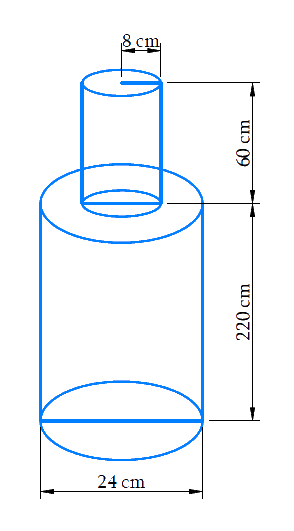Visually it’s clear that

Volume of the solid iron pole $$=$$ volume of larger cylinder $$+$$ volume of smaller cylinder

Mass of iron in the pole  $$=8\,\rm{g}$$ $$\;\times\;$$volume of the solid iron pole in $${\text{c}}{{\text{m}}^3}$$

We will find the volume of the solid by using formula;

Volume of the cylinder$$= \pi {r^2}h$$

where $$r$$ and $$h$$ are the radius and height of the cylinder respectively.

Steps:

Radius of larger cylinder,\begin{align}R = \frac{{24 \rm cm}}{2} = 12cm\end{align}

Height of larger cylinder,$$H = 220 \rm cm$$

Radius of smaller cylinder,$$r = 8 \rm cm$$

Height of smaller cylinder,$$h = 60 \rm cm$$

Volume of the solid iron pole $$=$$ volume of larger cylinder $$+$$ volume of smaller cylinder

\begin{align}&= \pi {R^2}H + \pi {r^2}h\\&= \pi \begin{pmatrix} 12 \rm cm \times 12cm \\ \times 220 \rm cm + 8cm \\ \times 8 \rm cm \times 60cm \end{pmatrix}\\&= 3.14 \times \!\! \begin{pmatrix} 31680 \rm c{m^3} \\ + 3840c{m^3} \end{pmatrix} \\&= 3.14 \times 35520 \rm c{m^3}\\&= 111532.8 \rm c{m^3}\end{align}

Mass of $$1 \rm cm^3$$ iron is $$\rm 8g$$

Mass of iron in the pole $$= 8 \rm g ×$$ volume of the solid iron pole in $$\rm cm^3$$

\begin{align}&= 8 \rm g \times 111532.8\\&= 892262.4g\\&= \frac{{892262.4}}{{1000}}kg\\&= 892.2624kg\end{align}

Mass of iron in the pole is $$892.26\,\rm{ kg}$$

## Chapter 13 Ex.13.2 Question 7

A solid consisting of a right circular cone of height $$120\,\rm {cm}$$ and radius $$60\rm\,{ cm}$$ standing on a hemisphere of radius $$60\rm\,{ cm}$$ is placed upright in a right circular cylinder full of water such that it touches the bottom. Find the volume of water left in the cylinder, if the radius of the cylinder is $$60\, \rm{cm}$$ and its height is $$180\,\rm {cm}.$$

### Solution

What is known?

A solid consisting of a right circular cone of height $$120\rm{cm}$$ and radius $$60\rm{cm}$$ standing on a hemisphere with the same radius is placed upright in a right circular cylinder of radius $$60\rm{cm}$$ and height $$180\rm{cm}$$, full of water such that it touches the bottom.

What is unknown?

Volume of water left in the cylinder.

Reasoning:

Draw a figure in accordance with the given information to visualize effectively.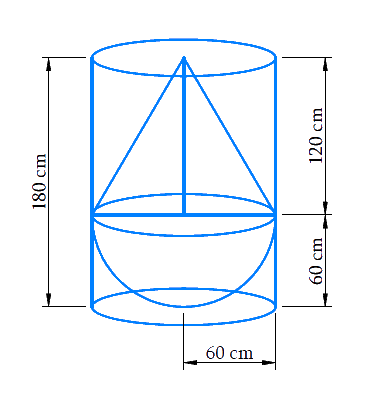From the figure, we observe that the solid fits totally into the cylinder as the height of the solid is same as the height of the cylinder

Since the cylinder was full of water before immersing the solid into it, so the entirespace in the cylinder is occupied by the solid and the left-over water.

Volume of the cylinder $$=$$ volume of the solid $$+$$ volume of the water left in the cylinder

Therefore,

Volume of the water left in the cylinder $$=$$ volume of the cylinder $$–$$ volume of the solid

Hence,

Volume of the water left in the cylinder $$=$$ volume of the cylinder $$–$$ volume of the hemisphere $$–$$ volume of the cone

We will find the volume of the solid by using formulae;

Volume of the hemisphere\begin{align} = \frac{2}{3}\pi {r^3}\end{align}

where $$r$$ is the radius of the hemisphere

Volume of the cone\begin{align} = \frac{1}{3}\pi {r^2}h\end{align}

where $$r$$ and $$h$$ are the radius and height of the cone respectively.

Volume of the cylinder $$= \pi {r^2}h$$

where $$r$$ and $$h$$ are radius and height of the cylinder respectively.

Steps:

Radius of hemispherical part $$=$$ Radius of conical part $$=$$ Radius of cylinder$$= r = 60 \rm cm$$

Height of the conical part of solid $$= h = 120 \rm cm$$

Height of the cylinder, $$= H = 180 \rm cm$$

Volume of the water left in the cylinder $$=$$ volume of the cylinder $$–$$ volume of the hemisphere $$–$$ volume of the cone

\begin{align}&= \pi {r^2}H - \frac{1}{3}\pi {r^2}h - \frac{2}{3}\pi {r^3}\\&= \frac{1}{3}\pi {r^2}\left( {3H - h - 2r} \right)\\&= \frac{1}{3} \times \frac{{22}}{7} \times 60 \rm cm \times 60cm \times\left( 3 \times 180 \rm cm -120 \rm cm - 2 \times 60 \rm cm \right) \\&= \frac{1}{3} \times \frac{{22}}{7} \times 60 \rm cm \times 60 \rm cm \times 300 \rm cm \\&= \frac{{7920000}}{7} \rm c{m^3}\\&= 1131426.57 \rm c{m^3}\\&= \frac{{1131426.57}}{{1000000}}\rm {m^3}\\&= 1.13142657 \rm {m^3}\end{align}

## Chapter 13 Ex.13.2 Question 8

A spherical glass vessel has a cylindrical neck $$8\rm\,{ cm}$$ long, $$2\rm\,{ cm}$$ in diameter; the diameter of the spherical part is $$8.5\rm\,{ cm}$$. By measuring the amount of water it holds, a child finds its volume to be $$345\rm\,{ cm^3}$$. Check whether she is correct, taking the above as the inside measurements, and $$\pi = 3.14.$$

### Solution

What is known?

A spherical glass vessel with a cylindrical neck of length $$=8\rm\,{ cm}$$ and diameter $$=2\rm\,{ cm}$$

The diameter of the spherical part $$=8.5\rm\,{ cm}$$

According to the child volume of the vessel $$345\rm{cm}^3$$

What is unknown?

Whether the child is correct in saying the volume of the glass vessel is $$345\,{\text{c}}{{\text{m}}^3}$$

Reasoning:

Draw a figure to visualize the glass vessel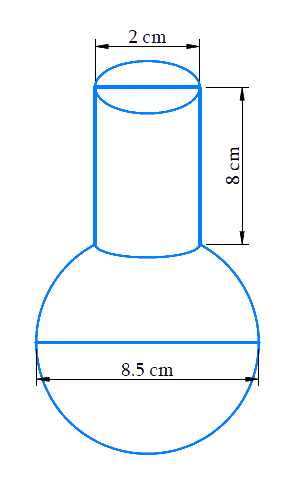Since the glass vessel is a combination of a sphere and a cylinder

Volume of glass vessel $$=$$ Volume of the spherical part $$+$$ Volume of the cylindrical part

Steps:

Height of cylindrical part,$$h = 8 \rm cm$$

Radius of cylindrical part,\begin{align}{r_1} = \frac{{2 \rm cm}}{2} = 1 \rm cm\end{align}

Radius of spherical part,\begin{align}{r_2} = \frac{{8.5 \rm cm}}{2} = 4.25 \rm cm\end{align}

Volume of the glass vessel $$=$$ volume of the spherical part $$+$$ volume of the cylindrical part

\begin{align}&= \frac{4}{3}\pi r_2^3 + \pi r_1^2h\\&= \pi \left( {\frac{4}{3}r_2^3 + r_1^2h} \right)\\ &= 3.14 \!\! \times \!\!\begin{pmatrix} \frac{4}{3} \times 4.25 \rm cm \\ \times 4.25 \rm cm \\ \times 4.25 \rm cm \\ + 1 \rm cm \\ \times 1 \rm cm \\ \times 8 \rm cm \end{pmatrix} \\&= \begin{Bmatrix} 3.14 \\\times \begin{pmatrix} 102.354 \rm c{m^3} \\ + 8 \rm c{m^3} \end{pmatrix} \end{Bmatrix} \\&= 3.14 \times 110.354 \rm c{m^3}\\&= 346.51 \rm c{m^3}\end{align}

Therefore, the child is not correct in saying that the volume of the glass vessel is $$345\rm{cm}^3$$